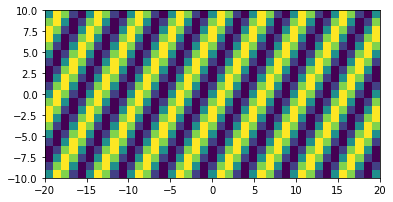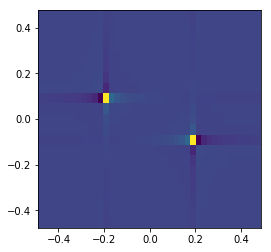# 2D Fourier transform¶

:

import numpy as np
import matplotlib.pyplot as plt

from deconvoluted import fourier_transform



Suppose we want to compute the 2D fourier transform $$F(p, q)$$ of a function $$f(x, y)$$. Let us generate some data which has a frequency of $$0.2$$ Hz in the $$x$$ direction, and $$0.1$$ Hz in the $$y$$ direction:

:

x = np.linspace(-20, 20, 41)
y = np.linspace(-10, 10, 21)
X, Y = np.meshgrid(x, y)
f_xy = np.sin(0.2 * 2 * np.pi * X + 0.1 * 2 * np.pi * Y)

plt.imshow(f_xy, extent=(x.min(), x.max(), y.min(), y.max()))
plt.show()Taking the transform is now simply a matter of calling fourier_transform:

:

F_pq, p, q = fourier_transform(f_xy, x, y)

:

plt.imshow(F_pq.real, extent=(p.min(), p.max(), q.min(), q.max()))
plt.show()We see two resonances, exactly where we would expect them!